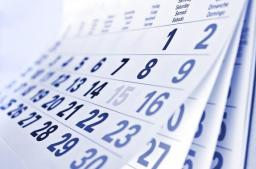# Determine 2250

The day number is the serial number of the day in the relevant month (i.e., the number on 5 August 2016 is 5). The digit sum of the day is the sum of the values ​​of all digits on the date of that day (i.e., the digit sum on 5 August 2016 is 5 + 8 + 2 + 0 + 1 + 6 = 22). A lucky day is one whose day number is equal to the digit of the day. Determine how many happy days there are in 2016 and which they are.

n =  10

### Step-by-step explanation:

20.9.2016
21.9.2016
22.9.2016
23.9.2016
24.9.2016
25.9.2016
26.9.2016
27.9.2016
28.9.2016
29.9.2016Did you find an error or inaccuracy? Feel free to write us. Thank you!

Tips for related online calculators
Do you want to convert time units like minutes to seconds?